Anzeige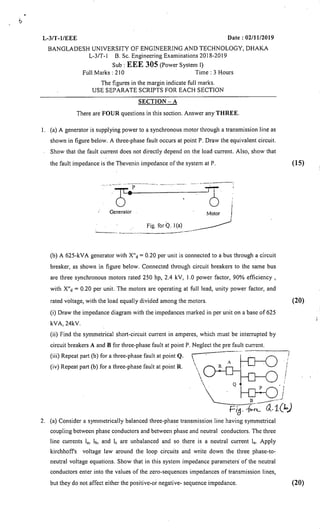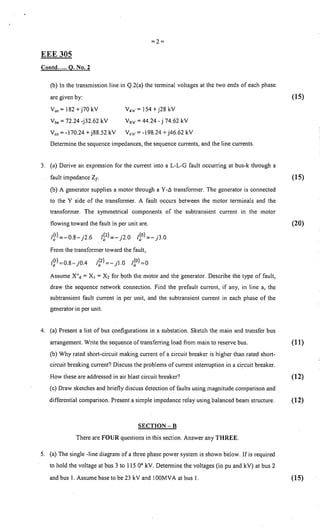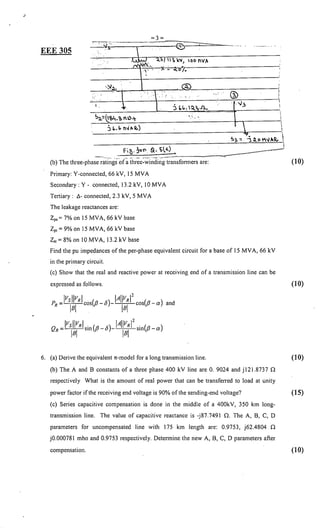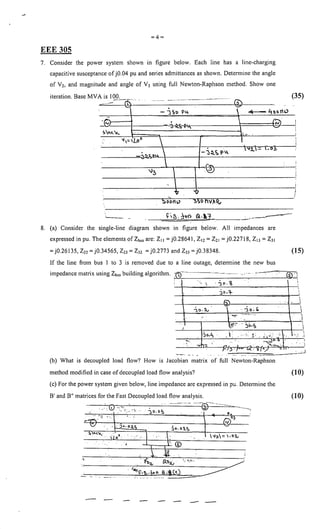Anzeige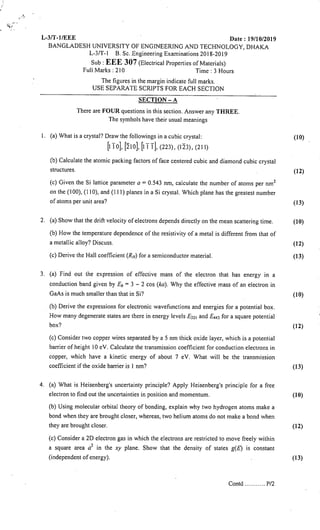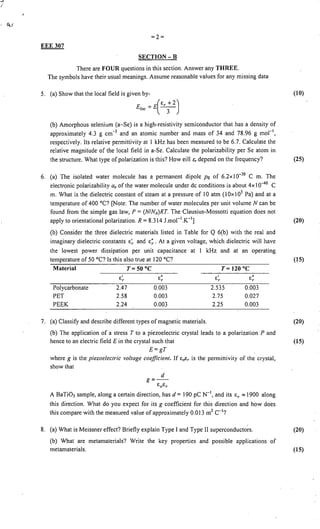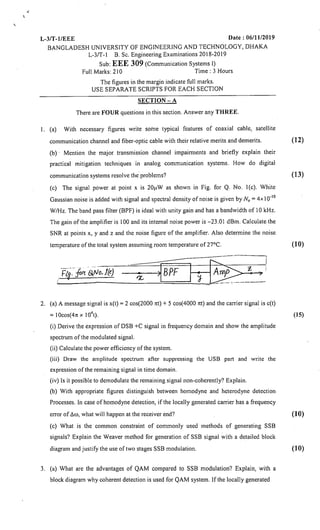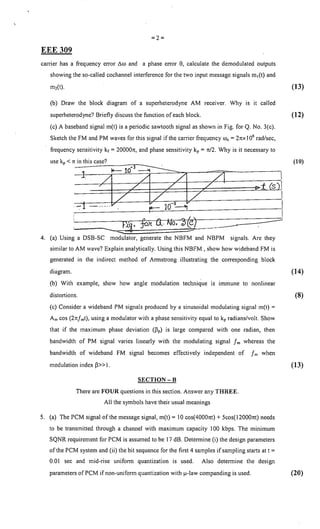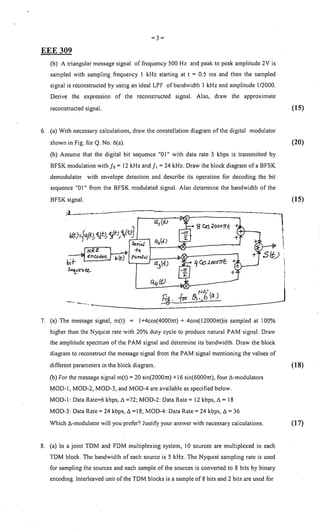Anzeige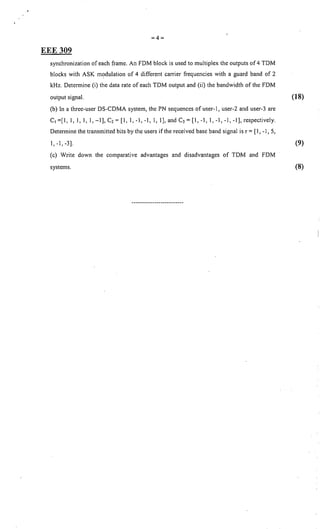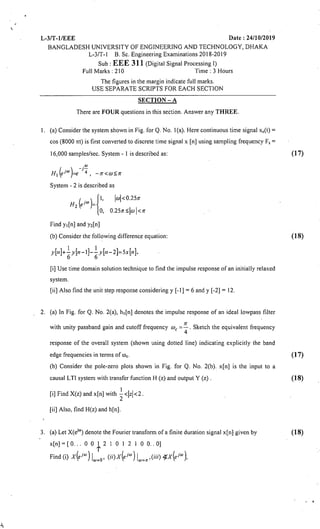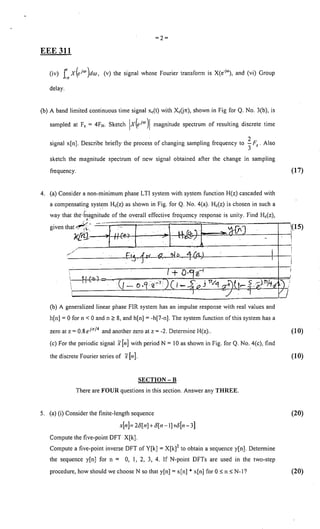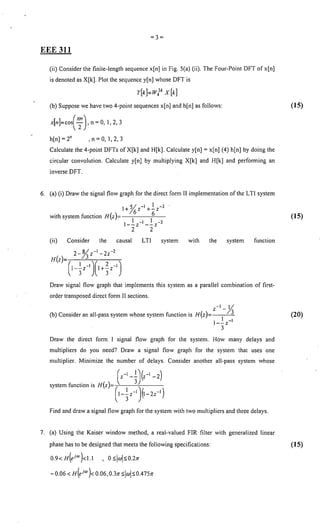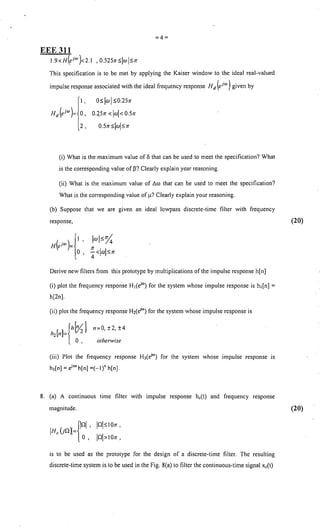Anzeige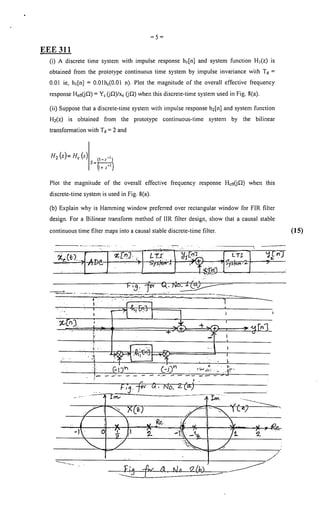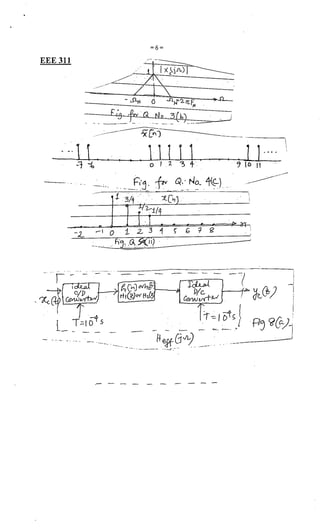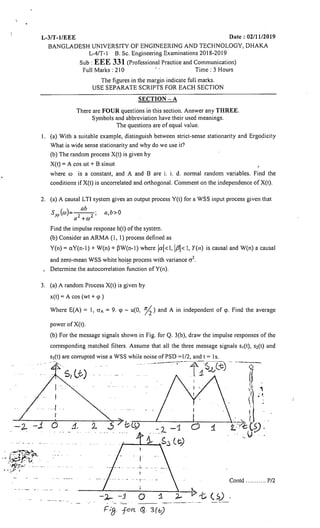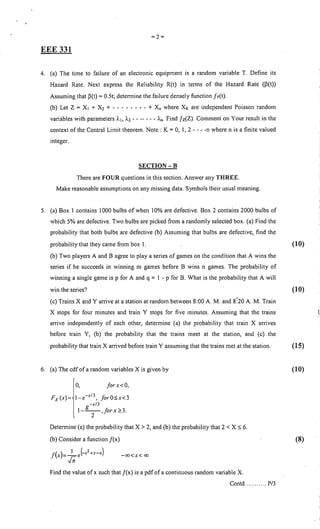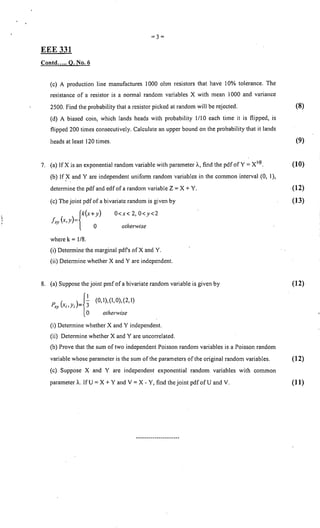Anzeige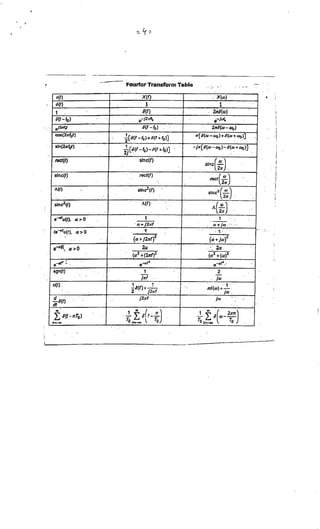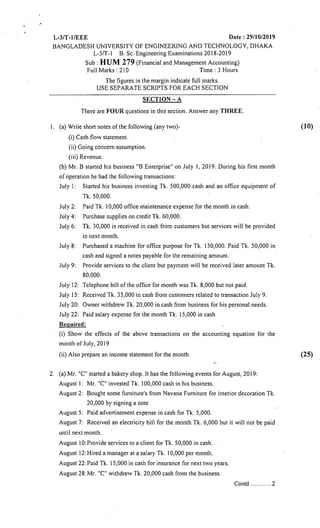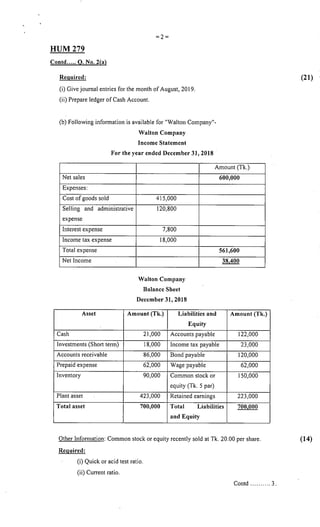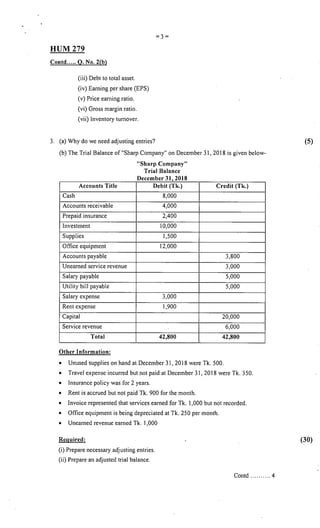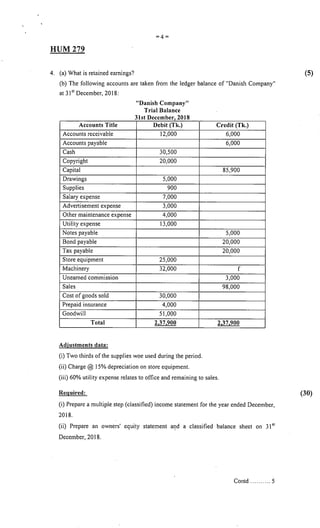Anzeige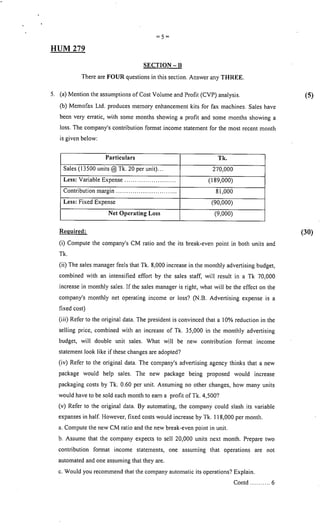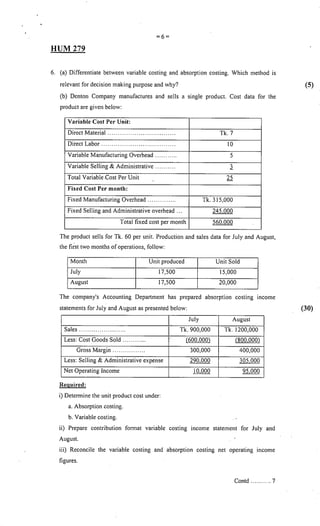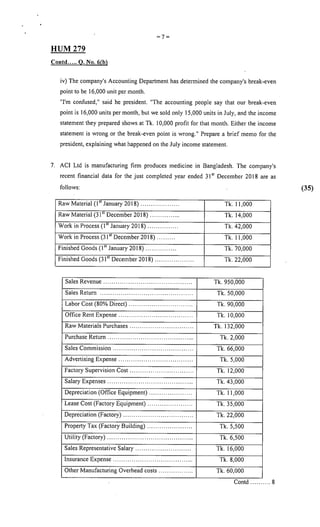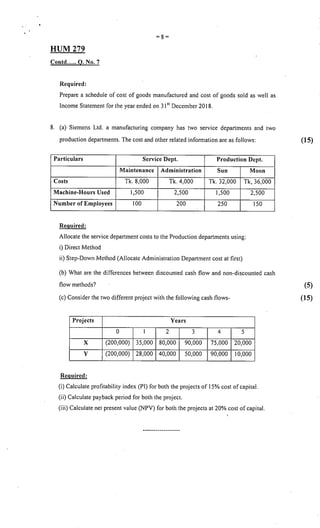Nächste SlideShare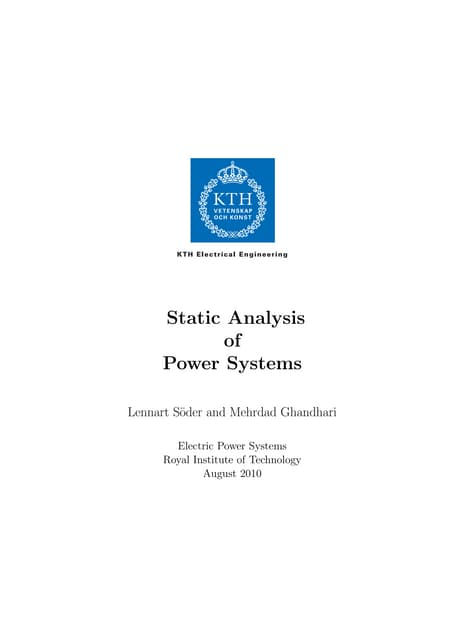Static analysis of power systems
1 von 28
Anzeige

### L 3,t-1,eee, 2018-2019

1. L-3/T-1/EEE Date: 02/1112019 BANGLADESH UNIVERSITY OF ENGINEERING AND TECHNOLOGY, DHAKA L-3/T-l B. Sc. Engineering Examinations 2018-2019 Sub: EEE 305 (Power System I) Full Marks: 210 Time: 3 Hours The figures in the margin indicate full marks. USE SEPARATE SCRIPTS FOR EACH SECTlON SECTION-A There are FOUR questions in this section. Answer any THREE. I. (a) A generator is supplying power to a synchronous motor through a transmission line as shown in figure below. A three-phase fault occurs at point P. Draw the equivalent circuit. Show that the fault current does not directly depend on the load current. Also, show that the fault impedance is the Thevenin impedance of the system at P. (15) Generator Fig. for Q. I (a) Motor (b) A 625-kVA generator with X"d = 0.20 per unit is connected to a bus through a circuit breaker, as shown in figure below. Connected through circuit breakers to the same bus are three synchronous motors rated 250 hp, 2.4 kV, 1.0 power factor, 90% efficiency, with X"d = 0.20 per unit. The motors are operating at full lead, unity power factor, and rated voltage, with the load equally divided among the motors. (i) Draw the impedance diagram with the impedances marked in per unit on a base of 625 kVA,24kV. (ii) Find the symmetrical short-circuit current in amperes, which must be interrupted by circuit breakers A and B for three-phase fault at point P. Neglect the pre fault current. (20) (iii) Repeat part (b) for a three-phase fault at point Q. (iv) Repeat part (b) for a three-phase fault at point R. , .' I i , P I B --J FiCJ' ¥:rL <1.1(1.,) 2. (a) Consider a symmetrically balanced three-phase transmission line having symmetrical coupling between phase conductors and between phase and neutral conductors. The three line currents la, Ib, and Ie are unbalanced and so there is a neutral current In. Apply kirchhoffs voltage law around the loop circuits and write down the three phase-to- neutral voltage equations. Show that in this system impedance parameters' of the neutral conductors entcr into the values of the zcro-sequences impedances of transmission lines, but they do not affect either the positivc-or negative- sequence impedance. (20)
2. =2= EEE 305 Contd ..... Q. NO.2 (b) In the transmission line in Q.2(a) the tenninal voltages at the two ends of each phase are given by: (15) Van= 182 +j70 kV Va'n'= 154 +j28 kV Vbn= 72.24 -j32.62 kV Vb'n'= 44.24 - j 74.62 kV Ven= -170.24 +j88.52 kV Ve'n'= -198.24 +j46.62 kV Determine the sequence impedances, the sequence currents, and the line currents. 3. (a) Derive an expression for the current into a L-L-G fault occurring at bus-k through a fault impedance Zj. (15) (b) A generator supplies a motor through a Y-t; transformer. The generator is connected to the Y side of the transformer. A fault occurs between the motor terminals and the transfonner. The symmetrical components of the subtransient current in the motor flowing toward the fault in per unit are. (20) 1~1)=-0.8- j2.6 1~2)=_ j2.0 I~O)=_j3.0 From the transfonner toward the fault, 1~1) =0.8-jO.4 1~2)=- jl.O I~o)=0 Assume X"d = XI = X2 for both the motor and the generator. Describe the type of fault, draw the sequence network connection. Find the prefault current, if any, in line a, the subtransient fault current in per unit, and the subtransient current in each phase of the generator in per unit. 4. (a) Present a list of bus configurations in a substation. Sketch the main and transfer bus arrangement. Write the sequence of transferring load from main to reserve bus. (11) (b) Why rated short-circuit making current of a circuit breaker is higher than rated short- circuit breaking current? Discuss the problems of current interruption in a circuit breaker. How these are addressed in air blast circuit breaker? (12) (c) Draw sketches and briefly discuss detection of faults using magnitude comparison and differential comparison. Present a simple impedance relay using balanced beam structure. (12) SECTION -B There are FOUR questions in this section. Answer any THREE. 5. (a) The single -line diagram of a three phase power system is shown below. 1f is required to hold the voltage at bus 3 to 115 0° kV. Determine the voltages (in pu and kV) at bus 2 and bus I. Assume base to be 23 kV and 100MVA at bus I. (15)
3. =3= (10) '- ~:, .. , y. '. ~~)/. , , , <;:.J~ @ ! , I ".' ., (}) , '" " ',.'- " ~ . " " '. "" ''»3 ; V ~ bI.. f.t...I'L I !>:l.=Q'Y-I. 'b l "',. ~ ;-, . 1 -j 1..1. n--ip,!l.) v -~-,,- (b) The three-phase ratingso(; thr;e=~~~di;;-g transfonners are: Primary: V-connected, 66 kV, 15 MVA Secondary: Y - connected, 13.2 kV, 10 MVA Tertiary: D,- connected, 2.3 kV, 5 MVA The leakage reactances are: Zps= 7% on 15 MVA, 66 kV base Zpt = 9% on 15 MVA, 66 kV base Zst = 8% on 10 MVA, 13.2 kV base Find the pu impedances of the per-phase equivalent circuit for a base of 15 MVA, 66 kV EEE 305 in the primary circuit. (c) Show that the real and reactive power at receiving end of a transmission line can be expressed as follows. (10) p JVsIIVRlcos(p_o)JAllvl cos(p-a) RIEl. lEI Q JVsIIVRlsin(p_o)JAllvl sin(p-a) R I~ I~ and 6. (a) Derive the equivalent 7t-model for a long transmission line. (10) (b) The A and S constants of a three phase 400 kV line are O. 9024 and j121.8737 n respectively What is the amount of real power that can be transferred to load at unity power factor if the receiving end voltage is 90% of the sending-end voltage? (c) Series capacitive compensation is done in the middle of a 400kV, 350 km long- transmission line. The value of capacitivc reactance is -j87.7491 n. The A, S, C, D parameters for uncompensated line with 175 km length are: 0.9753, j62.4804 n jO.000781 mho and 0.9753 respectively. Determine the new A, S, C, D parameters after (15) compensation. (10)
4. =4= EEE 305 J (35) • , ~noTl'" - "1 So Pl. l ~ :G_ I ~{'V} • -~*l.-l'U; ~ I " " ••'v... .. } ,'>'. • I 'I1=-ILoo •, 1" 1.'= r:-CS1 I . . -"1~~ PI.. ;).ll.r,~-I.. , '-I;') '.~ ~ , , I V. ~, 7. Consider the power system shown in figure below. Each line has a line-charging capacitive susceptance of jO.04 pu and series admittances as shown. Determine the angle of V2, and magnitude and angle of V3 using full Newton-Raphson method. Show one iteration. Base MV A is 100. -~o--G)---"--=--"'-~-'-----~--- , . '-- 8. (a) Consider the single-linc diagram shown in figure below. All impedances are expressed in pu. The elements oflbu, are: III = jO.28641, ll2 = lZI = jO.22718, l13 = l31 = jO.26135, l22 = jO.34565, l23 = l32 = jO.2773 and l33 = jO.38348. (15) J , ~ , I ',. ., "",}o. -:'l •• '- I I , I Q) . "10. ~ .. "",} o. " "".,0:':"' ......_.-:.. .' , , 1 "'j!"'''' .. ~o.~ " j •. ~ t '".' r:. Jo' I ~ :. ., ::' ., ~, "-'lr5~fo-:% , , ...;. Tic '. ""'l:;7,"",-_:, I J .. " " '--- If the line from bus I to 3 is removed due to a line outage, determine the new bus impedance matrix using lbu, building algorithm. ~~ @)~ , (b) What is decoupled load flow? How is Jacobian matrix of full Newton-Raphson method modified in case of decoupled load flow analysis? (c) For the power system given below, line impcdance are expressed in pu. Determine the (10) (10) .. '@ 11 . <9.1>~ Q ;tc.) ., B' and B" matrices for the Fast Decoupled load flow analysis. ',' (;):'.:",:.'. ... ' ~D.b':> ~_ .. _- --@~._--~- .'-":) ,.... '.. . .-_J.G; ~~. - ~ ' ... ':J',o,tr, :"I •• ot,:> ~~<~ .. iLo' - -
5. 1....•.•. L-3/T-lIEEE Date: 19/10/2019 BANGLADESH UNIVERSITY OF ENGINEERING AND TECHNOLOGY, DHAKA L-3/T-I B. Sc. Engineering Examinations 2018-2019 Sub: EEE 307 (Electrical Properties of Materials) Full Marks: 2 I0 Time: 3 Hours The figures in the margin indicate full marks. USE SEPARATE SCRIPTS FOR EACH SECTION SECTION -A There are FOUR questions in this section. Answer any THREE. The symbols have their usual meanings I. (a) What is a crystal? Draw the followings in a cubic crystal: (10) [ITo], , [IIT], (223), (123), (2 I I) (b) Calculate the atomic packing factors of face centered cubic and diamond cubic crystal structures. (12) (c) Given the Si lattice parameter a = 0.543 nm, calculate the number of atoms per nm2 on the (100), (I 10), and (I I 1) planes in a Si crystal. Which plane has the greatest number of atoms per unit area? (13) 2. (a) Show that the drift velocity of electrons depends directly on thc mean scattering time. (10) (b) How the tempcrature dependence of the resistivity of a metal is different from that of a metallic alloy? Discuss. (12) (c) Derive the Hall coefficient (RH) for a semiconductor material. (13) 3. (a) Find out the expression of effective mass of the electron that has energy in a conduction band given by Eb = 3 - 2 cos (ka). Why the effective mass of an electron in GaAs is much smaller than that in Si? (10) (b) Derive the expressions for electronic wavefunctions and energies for a potential box. How many degenerate states are there in energy Icvels E321 and E44] for a square potential box? (12) (c) Consider two copper wires separated by a 5 nm thick oxide layer, which is a potential barrier of height IDe V. Calculate the transmission coefficient for conduction electrons in copper, which have a kinetic energy of about 7 eV. What will be the transmission coefficient if the oxide barrier is I nm? (13) 4. (a) What is Heisenberg's uncertainty principle? Apply Heisenberg's principle for a free electron to find out the uncertainties in position and momentum. (10) (b) Using molecular orbital theory of bonding, explain why two hydrogen atoms make a bond when they are brought closer, whereas, two helium atoms do not make a bond when they are brought closer. (12) (c) Consider a 2D electron gas in which the electrons are restricted to move freely within a square area a2 in the xy plane. Show that the density of states geE) is constant (independent of energy). (13) Contd P/2
6. .' =2= EEE307 SECTION -B There are FOUR questions in this section. Answer any THREE. The symbols have their usual meanings. Assume reasonable values for any missing data 5. (a) Show that the local field is given by- (10) E = E(Er +2) lac 3 (b) Amorphous selenium (a-Se) is a high-resistivity semiconductor that has a density of approximately 4.3 g cm-3 and an atomic number and mass of 34 and 78.96 g mor l , respectively. Its relative permittivity at 1 kHz has been measured to be 6.7. Calculate the relative magnitude of the local field in a-Se. Calculate the polarizability per Se atom in the structure. What type of polarization is this? How eill &r depend on the frequency? (25) 6. (a) The isolated water molecule has a permanent dipole po of 6.2x I0-30 C m. The electronic polarizability ae of the water molecule under dc conditions is about 4x 10-40 C m. What is the dielectric constant of steam at a pressure of 10 atm (lOx 105 Pa) and at a temperature of 400°C? [Note: The number of water molecules per unit volume N can be found from the simple gas law, P = (N/NA)RT. The Clausius-Mossotti equation does not apply to orientational polarization. R = 8.314 J.morl.K-1 ] (20) (b) Consider the three dielectric materials listed in Table for Q 6(b) with the real and imaginary dielectric constants E~ and E;. At a given voltage, which dielectric will have the lowest power dissipation per unit capacitance at I kHz and at an operating temperature of 50°C? Is this also true at 120°C? (15) Material T= 50°C T= 120°C E' En E' En r r r r Polycarbonate 2.47 0.003 2.535 0.003 PET 2.58 0.003 2.75 0.027 PEEK 2.24 0.003 2.25 0.003 7. (a) Classify and describe different types of magnetic materials. (20) (b) The application of a stress T to a piezoelectric crystal leads to a polarization P and hence to an electric field E in the crystal such that (15) E=gT where g is the piezoelectric voltage coefficient. If EoEr is the permittivity of the crystal, show that d g=- EoEr A BaTi03 sample, along a certain direction, has d = 190 pC N-1 , and its Er '" 1900 along this direction. What do you expect for its g coefficient for this direction and how does this compare with the measured value of approximately 0.013 m2 C-I ? 8. (a) What is Meissner effect? Briefly explain Type I and Type II superconductors. (20) (b) What are metamaterials? Write the key properties and possible applications of metamaterials. (15)
7. ,..•~ L-3/T -1IEEE Date: 06/1112019 BANGLADESH UNIVERSITY OF ENGINEERING AND TECHNOLOGY, DHAKA L-3/T- I B. Sc. Engineering Examinations 2018-2019 Sub: EEE 309 (Communication Systems I) Full Marks: 2 I0 Time: 3 Hours The figures in the margin indicate full marks. USE SEPARATE SCRIPTS FOR EACH SECTION SECTION -A There are FOUR questions in this section. Answer any THREE. I. (a) With necessary figures write soine typical features of coaxial cable, satellite communication channel and fiber-optic cable with their relative merits and demerits. (b)' Mention the major transmission channel impairments and briefly explain their practical mitigation techniques in analog communication systems. How do digital communication systems resolve the problems? (c) The signal power at point x is 20~lW as shown in Fig. for Q. No. I(c). White Gaussian noise is added with signal and spectral density of noise is given by No = 4x 10- 10 W/Hz. The band pass filter (BPF) is ideal with unity gain and has a bandwidth of 10 kHz. The gain of the amplifier is 100 and its internal noise power is -23.01 dBm. Calculate the SNR at points x, y and z and the noise figure of the amplifier. Also determine the noise temperature of the total system assuming room temperature of 27°C. (12) (13) (10) (10) 2. (a) A message signal is x(t) = 2 cos(2000 ret) + 5 cos(4000 ret) and the carrier signal is c(t) = 1Ocos(4re x 10't). (15) (i) Derive the expression of DSB +C signal in frequency domain and show the amplitude spectrum of the modulated signal. (ii) Calculate the power efficiency of the system. (iii) Draw the amplitude spectrum after suppressing the USB part and write the exprcssion of the remaining signal in time domain. (iv) Is it possible to demodulate the remaining signal non-coherently? Explain. (b) With appropriate figures distinguish between homodyne and heterodyne detection Processes. In case of homodyne detection, if the locally generated carrier has a frequency crror of 600, what will happen at the receiver end? (c) What is the common constraint of commonly used methods of generating SSB signals? Explain the Weaver method for generation of SSB signal with a detailed block diagram and justify the use of two stages SSB modulation. 3. (a) What are the advantages of QAM compared to SSB modulation? Explain, with a block diagram why coherent detection is used for QAM system. If the locally generated (10)
8. =2= EEE 309 carner has a frequency error ""00 and a phase error 8, calculate the demodulated outputs showing the so-called cochannel interference for the two input message signals ml(t) and mz(t). (b) Draw the block diagram of a superheterodyne AM receIver. Why IS it called superheterodyne? Briefly discuss the function of each block. (c) A baseband signal met) is a periodic sawtooth signal as shown in Fig. forQ. No. 3(c). Sketch the FM and PM waves for this signal if the carner frequency We = 21tx106 rad/sec, frequency sensitivity kr = 200001t, and phase sensitivity kp = 1t/2. Why is it necessary to use kp < 1tin this case? -1-, __ 10-- 10- 3 --'1 __ -=-'_' .-"------.r-=-- 10-~ '----' __ -!i~. ~~ 4. (a) Using a DSB-SC modulator, generate the NBFM and NBPM signals. Are they similar to AM wave? Explain analytically. Using this NBFM , show how wideband FM is generated in the indirect method of Armstrong illustrating the corresponding block diagram. (b) With example, show how angle modulation technique IS Immune to nonlinear distortions. (c) Consider a wideband PM signals produced by a sinusoidal modulating signal met) = Amcos (21t/mt), using a modulator with a phase sensitivity equal to kp radians/volt. Show that if the maximum phase deviation (~p) is large compared with one radian, then bandwidth of PM signal varies linearly with the modulating signal 1m whereas the bandwidth of wideband FM signal becomes effectively independent of 1m when modulation index ~» 1. SECTION -8 There are FOUR questions in this section. Answer any THREE. All the symbols have their usual meanings 5. (a) The PCM signal of the message signal, met) = 10 cos(4000m) + 5cos(12000m) needs to be transmitted through a channel with maximum capacity 100 kbps. The minimum SQNR requirement for PCM is assumed to be 17 dB. Detennine (i) the design parameters of the PCM system and (ii) the bit sequence for the first 4 samples if sampling starts at t = 0.01 sec and mid-rise unifonn quantization is used. Also determine the design parameters ofPCM if non-uniform quantization with ~-law companding is used. (13) (12) (10) (14) (8) (13) (20)
9. =3= EEE 309 (b) A triangular message signal of frequency 500 Hz and peak to peak amplitude 2V is sampled with sampling frequency I kHz starting at t = 0.5 ms and then the sampled signal is reconstructed by using an ideal LPF of bandwidth I kHz and amplitude 1/2000. Derive the expression of the reconstructed signal. Also, draw the approximate reconstructed signal. (15) 6. (a) With necessary calculations, draw the constellation diagram of the digital modulator shown in Fig. for Q. No. 6(a). (20) (b) Assume that the digital bit sequence "01" with data rate 5 kbps is transmitted by BFSK modulation with 10= 12 kHz and 11= 24 kHz. Draw the block diagram of a BFSK demodulator with envelope detection and describe its operation for decoding the bit sequence "01" from the BFSK modulated signal. Also determine the bandwidth of the BFSK signal. (15) h(t)::'(l.ll~~ qJt~tift)) 1/f;;}] Sepio.l ~NRZ I 4 +0 bit- enCodeR. b(t) P«",{!L( S~e"l1ee.- o..l./tJ NO' Eg. -fnrr ~'Ib~C{) 7. (a) The message signal, met) = I+4cos(4000rrt) + 4cos(l2000rrt)is sampled at 100% higher than the Nyquist rate with 20% duty cycle to produce natural PAM signal. Draw the amplitude spectrum of the PAM signal and determine its bandwidth. Draw the block diagram to reconstruct the message signal from the PAM signal mentioning the values of different parameters in the block diagram. (b) For the message signal met) = 20 sin(2000m) +16 sin(6000m), four ~-modulators MOD-I, MOD-2, MOD-3, and MOD-4 are available as specified below. MOD-I: Data Rate=6 kbps, ~ =72; MOD-2: Data Rate = 12 kbps, ~ = 18 MOD-3: Data Rate = 24 kbps, ~ =18; MOD-4: Data Rate = 24 kbps, ~ = 36 Which ~-modulator will you prefer? Justify your answer with necessary calculations. 8. (a) In a joint TOM and FOM multiplexing system, 10 sources are multiplexed in each TOM block. The bandwidth of each source is 5 kHz. The Nyquist sampling rate is used for sampling the sources and each sample of the sources is converted to 8 bits by binary encoding. Interleaved unit of the TOM blocks is a sample of 8 bits and 2 bits are uscd for J (18) (17)
10. . =4= EEE 309 synchronization of each frame. An FOM block is used to multiplex the outputs of 4 TOM blocks with ASK modulation of 4 different carrier frequencies with a guard band of 2 kHz. Oetennine (i) the data rate of each TOM output and (ii) the bandwidth of the FOM output signal. (18) (b) In a three-user OS-COMA system, the PN sequences of user-I, user-2 and user-3 are C) =[1, I, I, I, 1, -1], Cz = [1,1, -1, -1,1,1], and C) = [I, -1,1, -1, -I, -I], respectively. Oetennine the transmitted bits by the users if the received base band signal is r = [1, -I, 5, 1,-1,-3]. (c) Write down the comparative advantages and disadvantages of TDM and FOM systems. (9) (8)
11. L-3fT-lIEEE Date: 24/10/2019 BANGLADESH UNIVERSITY OF ENGINEERING AND TECHNOLOGY, DHAKA L-3/T-I B. Sc. Engineering Examinations 2018-2019 Sub: EEE 311 (Digital Signal Processing I) Full Marks: 210 Time: 3 Hours The figures in the margin indicate full marks. USE SEPARATE SCRIPTS FOR EACH SECTION SECTION-A There are FOUR questions in this section. Answer any THREE. 1. (a) Consider the system shown in Fig. for Q. No. I(a). Here continuous time signal xc(t) = cos (8000 TIt)is first converted to discrete time signal x [n] using sampling frequency F, = 16,000 samples/sec. System - 1 is described as: .'" HI (e/"')=e-j4, -;r <OJ5,;r System - 2 is described as (17) H2 (e/'" )={I, 0, IOJI < 0.25;r 0.25;r 5,IOJ I<;r Find YI[n] and Y2[n] (b) Consider the following difference equation: 1 I y[n]+- y[n-I]-- y[n-2]=5x(n]. 6 6 [i] Use time domain solution technique to find the impulse response of an initially relaxed system. [ii] Also find the unit step response considering y [-I] = 6 and y [-2] = 12. 2. (a) In Fig. for Q. No. 2(a), hl[n] denotes the impulse response of an ideallowpass filter with unity passband gain and cutoff frequency OJe =;r . Sketch the equivalent frequency 4 response of the overall system (shown using dotted line) indicating explicitly the band edge frequencies in terms of Ole. (b) Consider the pole-zero plots shown in Fig. for Q. No. 2(b). x[n] is the input to a causal LTI system with transfer function H (z) and output Y (z) . [i] Find X(z) and x[n] with ~<lzl<2. 2 [ii] Also, find H(z) and h[n]. 3. (a) Let X(eiro ) denote the Fourier transform ofa finite duration signal x[n] given by x[n] = [ 0... 0 0 1 2 1 0 I 2 I 0 0.. 0] t Find (i) X(e/"') 1"'=0' (ii)X(e/"') 1"'=IT,(iii) ~X(e/"'), (18) (17) (18) (18) .. ,
12. =2= EEE 311 (iv) LX(eJw )dw, (v) the signal whose Fourier transform is X(e.j ",), and (vi) Group delay. (b) A band limited continuous time signal xc(t) with Xc(j1l), shown in Fig for Q. No. 3(b), is sampled at Fs = 4FN. Sketch IX(eJw)1 magnitude spectrum of resulting discrete time signal x[n). Describe briefly the process of changing sampling frequency to ~ Fs' Also 3 sketch the magnitude spectrum of new signal obtained after the change in sampling frequency. (17) [(15) , I 4. (a) Consider a non-minimum phase LTI system with system function H(z) cascaded with a compensating system Hc(z) as shown in Fig. for Q. No. 4(a). Hc(z) is chosen in such a way that the-inagnitude of the overall effective frequency response is unity. Find Hc(z), " :;~~- given tha~Jl3 jif:j ~// (b) A generalized linear phase FIR system has an impulse response with real values and h[n] = 0 for n < 0 and n <: 8, and h[n] = -h[7-n]. The system function of this system has a . /4 zero at z = 0.8 el" and another zero at z = -2. Determine H(z) .. (c) For the periodic signal X'[I1] with period N = 10 as shown in Fig. for Q. No. 4(c), find (10) the discrete Fourier series of X'[I1]. (10) SECTION -B There are FOUR questions in this section. Answer any THREE. 5. (a) (i) Consider the finite-length sequence X [11 ]= 28 + 8[11-]] +8[11- 3] Compute the five-point DFT X[k). Compute a five-point inverse DFT of Y[k] = X[kf to obtain a sequence y[n). Determine the sequence y[n] for n = 0, I, 2, 3, 4. If N-point DFTs are used in the two-step procedure, how should we choose N so that y[n] = x[n] • x[n] for 0 ::;n ::;N-l? (20) (20)
13. =3= EEE 311 (ii) Consider the finite-length sequenee x[n] in Fig. 5(a) (ii). The Four-Point DFT ofx[n] is denoted as X[k]. Plot the sequeneey[n) whose DFT is (b) Suppose we have two 4-point sequenees x[n) and h[n) as follows: X [11 ]= cos( ~1 ), n = 0, I, 2, 3 h[n) =2" , n=O, 1,2,3 Calculate the 4-point DFTs ofX[k) and H[k). Calculate y[n) = x[n) (4) h[n) by doing the circular convolution. Calculate y[n) by multiplying X[k) and H[k) and performing an inverse DFT. 6. (a) (i) Draw the signal flow graph for the direct form II implementation of the LTI system I 51 -I I -2 +/6z +-z with system function H(z )- I 16 1--z-I_-z-2 2 2 (ii) Consider the causal LTI system with the system function 2-%z-I_2z-2 H(z)- 3 (l-~z-I )(I+%Z-I) Draw signal flow graph that implements this system as a parallel combination of first- order transposed direct form 11sections. --I jj' (b) Consider an all-pass system whose system function is H(z)- ~ - 3 l-~z-I 3 Draw the direct foml I signal flow graph for the system. How many delays and multipliers do you need? Draw a signal flow graph for the system that uses one multiplier. Minimize the number of delays. Consider another all-pass system whose Find and draw a signal flow graph for the system with two multipliers and three delays. 7. (a) Using the Kaiser window method, a real-valued FIR filter with generalized linear phase has to be designed that meets the following specifications: 0.9< H(eif• )<I.l , 0 :5lwl:50.2;r - 0.06 < H(eiOJ )<0.06, 0.3;r :5lwl:50.4 75;r (15) (15) (20) (15)
14. =4= EEE 311 1.9<H(eiw)<2.1 , 0.5251T~lwl~1T This specification is to be met by applying the Kaiser window to the ideal real-valued impulse response associated with the ide'al frequency response Iid (eiw ) given by 1 , Iid(eiw)= 0, 2 , O~ Iw 1~0.251T 0.451T < Iwl< 0.51T 0.51T~lwl~1T (i) What is the maximum value of Ii that can be used to meet the specification? What is the corresponding value of~? Clearly explain year reasoning. (ii) What is the maximum value of I10J that can be used to meet the specification? What is the corresponding value of f!? Clearly explain your reasoning. (b) Suppose that we are given an ideal lowpass discrete-time filter with frequency response, (20) Iwl~~ , IT <lwl~1T 4 Derive new filters from this prototype by multiplications of the impulse response h[n] (i) plot the frequency response HI(ei"') for the system whose impulse response is hl[n] = h[2n). (ii) plot the frequency response H2(ei"') for the system whose impulse response is [ 1 { hbi 1 n=O, :t2,:t4 h2 n = o , olhenl/;se (iii) Plot the frequency response H3( ei"') for the system whose impulse response IS h3[n] = ein"h[n] =(_1)" h[n). 8. (a) A continuous time filter with impulse response hc(t) and frequency response magnitude. (20) I~*IOIT, 101>101T, is to be used as the prototype for the design of a discrete-time filter. The resulting discrete-time system is to be used in the Fig. 8(a) to filter the continuous-time signal xc(t)
15. =5= EEE 311 (i) A discrete time system with impulse response h1[n) and system function H,(z) is obtained from the prototype continuous time system by impulse invariance with Td = 0.01 ie, h1[n) = O.Olhc(O.OI n). Plot the magnitude of the overall effective frequency response Hcn{jO) = Yc(jO)/xc (jO) when this discrete-time system used in Fig. 8(a). (ii) Suppose that a discrete-time system with impulse response h2[n) and system function I-h(z) is obtained from the prototype continuous-time system by the bilinear transformation with Td = 2 and Plot the magnitude of the overall effective frequency response Hcn{jO) when this discrete-time system is used in Fig. 8(a). (b) Explain why is Hamming window preferred over rectangular window for FIR filter design. For a Bilinear transform method of IIR filter design, show that a causal stable continuous time filter maps into a causal stable discrete-time filter. (15) - i" , ';I~_~- Re. --~,: ' ~1r~1. L T:L i +. fys1em- 2~ ..:~r!':1J.:-. - ,~ " ~~~~ -" . --;--""?~~-=" ~""'~";""""""-~-----' ~'l -.-- .!- X [nJ. ",," L7:/ .. ,,' ~,..{,;'. >r~I~ "" 6 -I , ---L _ 59~__ _~Jt .', I -----r--,"j--f. ~.D:"2la:) . fm.- .. ----- 1 , .. , , *,...~ '. " '. , , t V:,J I , I JL;[n} , • 't,. , , ' -t~ .:r", . :tjlriJ_ .• =/' .:t: ;. l' ..! I " I .. i: "-. ~':"'t~ 'e, ..- I - ".1 ..""~~,," I.' :' '7f' , (~, I. ,
16. =6= EEE 311 ,"lJ --', t' ~ I . -1"::b illlJ~~.---., ,11 .... O ,. 2 -:z A.' - :J T CJ Ib 11 ... ~-"..- .-' -L ..--1 0 i 2- .3 '1 ~ G 1- I? . FJ~.~910;). - - --' .._- r ~ - - ---l7 I ,~~~~ L T~IO'1'5 - _. -- ~ICv,J 0I!-J' . t71(C) err /'h,(c -------
17. L-31T-1/EEE Date: 02/11/2019 BANGLADESH UNIVERSITY OF ENGINEERING AND TECHNOLOGY, DHAKA L-41T-l B. Sc. Engineering Examinations 2018-2019 Sub: EEE 331 (Professional Practice and Communication) Full Marks: 210 Time: 3 Hours The figures in the margin indicate full marks. USE SEPARATE SCRIPTS FOR EACH SECTION SECTION-A There are FOUR questions in this section. Answer any THREE. Symbols and abbreviation have their used meanings. The questions are of equal value. I. (a) With a suitable example, distinguish between strict-sense stationarity and Ergodicity What is wide sense stationarity and why do we use it? (b) The random process X(t) is given by X(t) = A cos rot + B sinrot c where ro is a constant, and A and Bare i. i. d. normal random variables. Find the conditions if X(t) is uncorrelated and orthogonal. Comment on the independence of X(t). 2. (a) A causal LTI system gives an output process yet) for a WSS input process given that Syy(w)= 2 ab 2; a,b>O a +w Find the impulse response h(t) of the system. (b) Consider an ARMA (1, I) process defined as yen) = uY(n-l) + Wen) + ~W(n-I) where lal<l, IfJl<I, yen) is causal and Wen) a causal and zero-mean WSS white hoi~e process with variance a2 . Determine the autocorrelation function ofY(n). 3. (a) A random Process X(t) is given by x(t) = A cos (wt + <p) Where E(A) = I, aA = 9. <p - u(O, ~) and A in independent of <po Find the average power of X(t). , I o -./_ -1 1- \$:> (t) (b) For the message signals shown in Fig. for Q. 3(b), draw the impulse responses of the corresponding matched filters. Assume that all the three message signals Sl(t), S2(t) and sJ(t) are corrupted wise a WSS while noise of PSD =1/2, and t = 1s. ... ~. tJ.. S.)",- C .t)- .1 r -j 0 :1. 2.. Contd P/2 -2- -j 0 1 .2--1> 1; (s) - .._.- --- -----~------ -~ Fla. fcJrt. ~. 3(6)
18. =2= EEE 331 4. (a) The time to failure of an electronic equipment is a random variable T. Define its Hazard Rate. Next express the Reliability R(t) in terms of the Hazard Rate (P(t)) Assuming that P(t) = 0.5t; determine the failure densely function h(t). (b) Let Z = Xl + X2 + - - - - - - - - + Xn where XK are independent Poisson random variables with parameters AI, 1'2 - - -- - - - I'n' Find Jz(Z). Comment on Your result in the context of the Central Limit theorem. Note: K = 0, I, 2 - - - -n where n is a finite valued integer. SECTION-B There are FOUR questions in this section. Answer any THREE. Make reasonable assumptions on any missing data. Symbols their usual meaning. 5. (a) Box 1 contains 1000 bulbs of when 10% are defective. Box 2 contains 2000 bulbs of which 5% are defective. Two bulbs are picked from a randomly selected box. (a) Find the probability that both bulbs are defective (b) Assuming that bulbs are defective, find the probability that they came from box I. (10) (b) Two players A and B agree to playa series of games on the condition that A wins the series if he succceds in winning m games before B wins n games. The probability of winning a single game is p for A and q = I - P for B. What is the probability that A will -oo<x< 00 win the series? (c) Trains X and Y arrive at a station at random between 8:00 A. M. and 8:20 A. M. Train X stops for four minutes and train Y stops for five minutes. Assuming that the trains arrive independently of each other, detennine (a) the probability that train X arrives before train Y, (b) the probability that the trains meet at the station, and (c) the probability that train X arrived before train Y assuming that the trains met at the station. 6. (a) The cdf of a random variables X is given by 0, jorx<O, Fx(x)= l_e-xI3, jorO:5,x<3 -x13 1-- g --,jorx?3. 2 Determine (a) the probability that X > 2, and (b) the probability that 2 < X:5, 6. (b) Consider a function f(x) j() 1 (_x2 +x-a) x=.,;;e Find the value ofx such that f(x) is a pdfofa continuous random variable X. Contd P/3 (10) (15) (10) (8)
19. =3= EEE 331 Contd ..... Q. NO.6 (c) A production line manufactures 1000 orun resistors that have 10% tolerance. The resistance of a resistor is a normal random variables X with mean 1000 and variance 2500. Find the probability that a resistor picked at random will be rejected. (d) A biased coin, which lands heads with probability 1/10 each time it is flipped, is flipped 200 times consecutively. Calculate an upper bound on the probability that it lands heads at least 120 times. 7. (a) If X is an exponential random variable with parameter A, find the pdf ofY = XI/p. (b) If?< and Yare independent uniform random variables in the common interval (0, I), determine the pdf and edf of a random variable Z = X + Y. (c) The joint pdf of a bivariate random is given by (8) (9) (10) (12) (13) { k(X+ y) fxy (x,y)= 0 O<X< 2, 0<y<2 otherwise where k = 1/8. (i) Determine the marginal pdfs of X and Y. (ii) Determine whether X and Yare independent. 8. (a) Suppose the joint pmf of a bivariate random variable is given by P (" I.)=j.!.. (0,1),(1,0),(2,1) xy X,,), 3 o otherwise (12) (i) Determine whether X and Y independent. (ii) Determine whether X and Yare uncorrelated. (b) Prove that the sum of two independent Poisson random variables is a Poisson random variable whose parameter is the sum of the parameters of the original random variables. (12) (c) Suppose X and Yare independent exponential random variables with common parameter A. IfU = X + Y and V = X - Y, find the joint pdf ofU and V. (11)
20. . -:. " . ,.;. ", x(11 X(f) " X{",! 6(11 1 1 1 B(f) 2Jr6(1l/) B(I-Io) 8-J2~n. 9-J~ , "J2JrfJ 6(1 '01 266(", -"'Il) cos(2"'011 1 ' 1T[6{", -wal +6{",.., "'ril] 2[6(1 -lol+6{f +fol] sln(21ffo'l 1 ' " - fir[ 5{", --war-5{", + "'01] -[ 6(f -fo)-cl(f +(01] 2J " , rect(l) slnc(f) sine(- "'J 2" " slnc(l) roc/(f) mct(::r) ,A{II '. slnc2(f) Slnc2(~) . " slnc2(/) A(f) A(::r ) o-alu(/), «>0 1 1 ,-- .a+ 121ff a+J(J)." .: '. /o-o1U(I), a>O f ' , . 1, ,. (a +f21f()2 (ti+ J",)2 e-a~1 a>O 2•• .' 2a " (a2 +(2Jrf)2 (a2 +(",)2 o-Irt' :, . e-af' e-af~:; sgn(t) 1 2 f"f J'" U(I) . , 1 1 1. "26(f)+ rhrt ".6(",) +-. ' I'" . ; 6(t) rbrl I'" " ' - •• , .1i:if~-!?-) 1'" ( 28) LB(I-oro) r;L 6 "'- r.n r. '}'; "- . 0 nc-oD 0 o n::.--co 0 . .' , I I, I I , II I